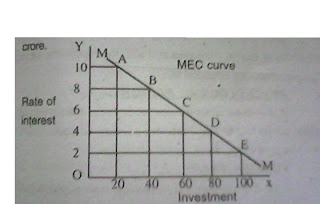### Ticker

6/recent/ticker-posts

# Marginal Efficiency of Capital and its factors

### Marginal Efficiency of Capital and its factorsThe volume of investment depend upon the following two factors :

1. Rate of interest.
2. Marginal Efficiency of Capital.

Before investing the money a businessman compares interest with the rate of marginal efficiency capital.If they expect that rate of profit will be greater than the rate of interest, then they invest the money otherwise not. For example the rate of interest is 15% and expected rate of profit is 18% then he will do the investment. Because it is more than the 15%.

MARGINAL EFFICIENCY OF CAPITAL

Keynes says, " The marginal efficiency of capital is equal to that rate of discount which would make the present value of the series of annuities given by the returns expected from the capital asset during its life just equal to its supply price."

The expected rate of return on capital is called the marginal efficiency of capital. In other words, marginal efficiency of capital is a return on investment which is based partly on expectations of future yields and partly on the actual price of the capital good concerned.
Actual price is the price which just includes the producer of capital good to produce a new capital goods. A producer will produce the machine if a buyer is ready to pay actual price. Actual price or inducement price is the same thing.
The marginal efficiency of capital depends upon two factors :

1. Expected Net return on an Asset.
2. Replacement Cost or Supply price of the asset .

EXPECTED RETURN OF AN ASSET :- The expected net return on an asset is that income which a person receives after selling the product produced by the machine or asset , If the expected rate of return is greater than the expenses made on it, then investment will increase

REPLACEMENT COST OR SUPPLY PRICE :- The marginal efficiency of capital will increase other things remaining the same, if the supply price or the price of the machines falls. On the other hand it falls with the increase in the supply price.

MEC = Expected yearly income/Supply price X 100

Increase in consumptions, leads to an increase in marginal efficiency of capital and investment. Investment will tend to increase so long as the marginal efficiency of capital is more than the prevailing rate of interest. In simple words investment tends to increase upto that point where marginal efficiency of capital is equal to the rate of interest.
We can explain it by the following table :EXPLANATION :- According to this schedule we assume that rate of interest in the market is 6% which is fixed.The table shows that as the volume of investment increases the marginal efficiency of capital falls.The rate of interest and MEC both are equal to each other when the investment volume is 6 crore.EXPLANATION :- This diagram shows that when investment is RS. 6 crore the marginal efficiency of capital and rate of interest both are equal to each other ( 6% ). So it is clear that rate of interest and marginal efficiency of capital both determine the volume of investment.

FACTORS WHICH AFFECT THE MARGINAL EFFICIENCY OF CAPITAL

1. DEMAND OF GOODS :- If the demand is greater than the marginal efficiency of capital will also be greater. On the other hand if the demand of goods is smaller then the marginal efficiency of capital will also be smaller.

2. PRICE OF COMMODITIES :- With the increase in the prices of goods, marginal efficiency of capital increases and with the fall in price the marginal efficiency of capital also falls.

3. COST OF PRODUCTION :- With the fall in the cost of production marginal efficiency of capital increases and with the rise in the cost, marginal efficiency of capital falls.

4. PSYCHOLOGICAL FACTOR :- If the businessman are optimistic, efficiency of capital will be higher and if they are pessimistic about future then marginal efficiency of capital will be low.

5. FOREIGN TRADE :- If the export demand increases, then producer will increase the investment because marginal efficiency of capital increases.

6. THE QUANTITY OF CAPITAL GOODS :- If the capital goods are already in the large quantity to meet the demand of the market, then it will not be beneficial for the investors to invest the money in the project and the marginal efficiency of capital will fall.

7. RATE OF POPULATION GROWTH :- If the rate of population growth is high then the demand of various goods will increase . So it will increase the marginal efficiency of capital. It falls with the slow bith rate.

8. TECHNOLOGICAL ADVANCEMENT :- Inventions and technological improvement encourages investment in various projects.So marginal efficiency of capital increases.

9. RATE OF TAXES :- If government imposes the taxes on various goods, it will increase the cost of production and will reduce the profit . So with the fall in profit the rate of investment and marginal efficiency of capital both falls.

10. LABOUR EFFICIENCY :- Efficiency of labour increases the marginal efficiency of capital lowers.

11. GOVERNMENT INTERFERENCE :- If the government interferes in the private business and imposes some restrictions then people will hesitate to invest and marginal efficiency of capital falls. On the other hand if it encourages the private business then marginal efficiency of capital will increase